# BITSAT Logical Reasoning Test - 3

## 10 Questions MCQ Test BITSAT Mock Tests Series & Past Year Papers | BITSAT Logical Reasoning Test - 3

Description
Attempt BITSAT Logical Reasoning Test - 3 | 10 questions in 10 minutes | Mock test for JEE preparation | Free important questions MCQ to study BITSAT Mock Tests Series & Past Year Papers for JEE Exam | Download free PDF with solutions
QUESTION: 1

Solution:
QUESTION: 2

Solution:
QUESTION: 3

### Group the following figures into three classes on the basis of identical properties.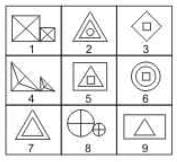Solution:

1,4,8 contain similar elements (not equal in size) each divided into four parts and attached to each other.
2,5,6 contain three elements (two of which are similar) plased one inside the other.
3,7,9 contain one element inside the other, which may or may not be similar.

QUESTION: 4

Find the Missing Number.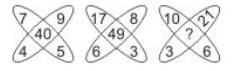Solution:

In the 1st fig
7 + 9 + 5 + 4 = 25
middle no 40 = 25 + 15
In the 2nd fig
17 + 8 + 3 + 6 = 34
middle no 49 = 34 + 15
In the 3rd fig
10 + 21 + 6 + 3 = 40
middle no x = 40 + 15 = 55 = correct answer

QUESTION: 5

In this letter series, some of the letters are missing. Choose the correct letter given below -
_ _ aba _ _ ba _ ab

Solution:

The series is ab / ab / ab / ab / ab / ab
Thus, the pattern ab is repeated

QUESTION: 6

Find out the term in the number series which is wrong.
1, 2, 5, 10, 17, 28

Solution: (0²+1),(1²+1),(2²+1),(3²+1),(4²+1). So 26 should be instead of 28.
QUESTION: 7

Select the correct mirror image of the figure (X) from amongst the given alternatives.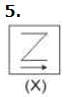Solution:
QUESTION: 8

Select the correct mirror image of the figure (X) from amongst the given alternatives.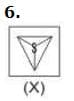Solution:
QUESTION: 9

Each of the following questions consists of five Problem figures marked 1,2,3,4 and 5 followed by five Answer figures marked A,B,C,D and E . Select a figure from the Answer figures which will continue the same series as given in the Problem figures.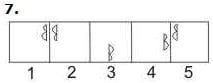Solution:
QUESTION: 10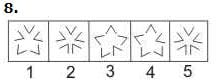Use Code STAYHOME200 and get INR 200 additional OFF Use Coupon Code